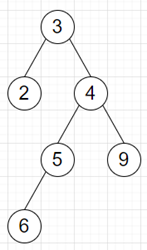# Program to find length of longest consecutive path of a binary tree in python

Suppose we have a binary tree; we have to find the longest path in the binary tree.

So, if the input is likethen the output will be 5 as longest consecutive sequence is [2, 3, 4, 5, 6].

To solve this, we will follow these steps −

• if root is null, then
• return 0
• maxPath := 0
• Define a function helper() . This will take node
• inc := 1, dec := 1
• if left of node is not null, then
• [left_inc, left_dec] := helper(left of node)
• otherwise,
• [left_inc, left_dec] := [0, 0]
• if right of node is not null, then
• [right_inc, right_dec] := helper(right of node)
• otherwise,
• [right_inc, right_dec] := [0, 0]
• if left of node is not null and value of node - value of left of node is same as 1, then
• inc := maximum of inc and (left_inc + 1)
• otherwise when left of node is not null and value of node - value of left of node is same as -1, then
• dec := maximum of dec and (left_dec + 1)
• if right of node is not null and value of node - value of right of node is same as 1, then
• inc := maximum of inc and (right_inc + 1)
• otherwise when right of node is not null and value of node - value of right of node is same as -1, then
• dec := maximum of dec and (right_dec + 1)
• if left of node is not null and right of node is not null and value of left of node - value of node is same as 1 and value of node - value of right of node is same as 1, then
• maxPath := maximum of maxPath and (left_dec + right_inc + 1)
• otherwise when left of node node is not null and right of node is not null and value of left of node - value of node is same as -1, then
• maxPath := maximum of maxPath and (left_inc + right_dec + 1)
• maxPath := maximum of maxPath, inc and dec
• return inc, dec
• From the main method do the following:
• helper(root)
• return maxPath

Let us see the following implementation to get better understanding −

## Example

Live Demo

class TreeNode:
def __init__(self, data, left = None, right = None):
self.val = data
self.left = left
self.right = right

def print_tree(root):
if root is not None:
print_tree(root.left)
print(root.val, end = ', ')
print_tree(root.right)

class Solution:
def solve(self, root):
if not root:
return 0
self.maxPath = 0

def helper(node):
inc, dec = 1, 1
if node.left:
left_inc, left_dec = helper(node.left)
else:
left_inc, left_dec = 0, 0
if node.right:
right_inc, right_dec = helper(node.right)
else:
right_inc, right_dec = 0, 0

if node.left and node.val - node.left.val == 1:
inc = max(inc, left_inc + 1)
elif node.left and node.val - node.left.val == -1:
dec = max(dec, left_dec + 1)

if node.right and node.val - node.right.val == 1:
inc = max(inc, right_inc + 1)
elif node.right and node.val - node.right.val == -1:
dec = max(dec, right_dec + 1)

if (node.left and node.right and node.left.val - node.val == 1 and node.val - node.right.val == 1):
self.maxPath = max(self.maxPath, left_dec + right_inc + 1)
elif (node.left and node.right and node.left.val - node.val == -1
and node.val - node.right.val == -1):
self.maxPath = max(self.maxPath, left_inc + right_dec + 1)

self.maxPath = max(self.maxPath, inc, dec)
return inc, dec

helper(root)
return self.maxPath

ob = Solution()
root = TreeNode(3)
root.left = TreeNode(2)
root.right = TreeNode(4)
root.right.left = TreeNode(5)
root.right.right = TreeNode(9)
root.right.left.left = TreeNode(6)
print(ob.solve(root))

## Input

root = TreeNode(3)
root.left = TreeNode(2)
root.right = TreeNode(4)
root.right.left = TreeNode(5)
root.right.right = TreeNode(9)
root.right.left.left = TreeNode(6)

## Output

5